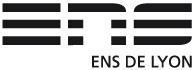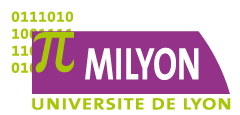17-20 juin 2013

Département de Mathématiques de l'ENS Lyon

Organisateurs : V. Pilloni (CNRS, ENS Lyon), B. Stroh (CNRS, P13), J. Tilouine (U. P13)

Cette conférence se tiendra du lundi 17 au jeudi 20 juin 2013 dans l'Amphi A de l'ENS Lyon.

Programme

Lundi 17 Juin

9h30-10h30 : L. Berger (ENS Lyon): Sen theory and locally analytic vectors

Résumé :

11h-12h : E. Hellman (U. Paris 6) : Density of potentially Barsotti-Tate representations

Résumé : Let K be a finite extension of Q_p. We prove that the Galois representations that become Barsotti-Tate after an abelian extension are Zariski-dense in the generic fiber of the universal deformation ring of an absolutely irreducible 2-dimensional residual Galois representation. The proof uses a map from an eigenvariety to the space of trianguline representations and a related density statement on the eigenvariety as a global input. This is joint work with Benjamin Schraen.

14h-15h : F. Brumley (U. Paris 13) : The work of Shin-Templier on fields of rationality of automorphic representations

Résumé : In a recent preprint, Shin and Templier investigate several inter-related questions on rationality properties of automorphic representations. Among the many beautiful results they prove, two are singled out as main theorems. One is in the spirit of Faltings' finiteness theorem on abelian varieties: there are only finitely many discrete automorphic representations (on GL(n) or a quasi-split classical group over a number field) with a given infinitesimal character, given ramification support, and bounded rationality field. The other is a generalization of a result of Serre to quasi-split classical groups: within a family of cohomological cusp forms whose level gets large, those with bounded rationality field are of density zero. The proofs of these results rely on Arthur's endoscopic transfer and build upon important work of Buzzard-Gee, Clozel, Serre, as well as the previous work of the authors on equidistribution in families of automorphic forms. Along the way, they formulate an extension of the Fontaine-Mazur conjecture in terms of integrality properties of Weil-Deligne representations associated to Galois representations. This talk will be a survey of their work.

15h30-16h30 : D. Geraghty (IAS, Princeton) : Modularity lifting beyond the "numerical coincidence" of Taylor and Wiles, I

Résumé : I will discuss my joint work with Frank Calegari which proves conditional modularity lifting theorems in situations where the "classical" method of Taylor and Wiles breaks down. Such situations include the case of self-dual geometric Galois representations over a totally real field with repeated Hodge--Tate weights and the case of geometric Galois representations over a general number field. The main theorems are conditional on conjectures asserting the existence of Galois representations over integral Hecke algebras and conjectures asserting certain cohomology vanishing results.

Mardi 18 Juin

9h00-10h00 : F. Herzig (U. Toronto) : On the classification of irreducible mod p representations of p-adic reductive groups

Résumé : I will report on the classification of irreducible admissible mod p representations of p-adic reductive groups in terms of supersingular representations. This is joint work in progress with N. Abe, G. Henniart, and M.-F. Vigneras.

10h15-11h15 : G. Rosso (U. Paris 13 et KU Leuven) : Formes modulaires quasi surconvergentes et zéros triviaux de la fonction L p-adique du carré symétrique

Résumé : Soit $f$ une forme modulaire de poids $k$ et Steinberg en $p$. Sous certaines hypoth\`eses sur le conducteur de $f$, on donne, par la m\'ethode de Greenberg et Tilouine, une formule pour la d\'eriv\'ee en $s=k-1$ de la fonction $L$ $p$-adique pour le carr\'e sym\'etrique de $f$, d\'emontrant ainsi une conjecture de Benois. \\ Crucial pour la d\'emonstration sont les formes modulaires quasi surconvergentes introduites par Skinner et Urban, dont on rappellera les propri\'et\'es principales.

11h30-12h30 : D. Geraghty : Modularity lifting beyond the "numerical coincidence" of Taylor and Wiles, II

14h00-15h00 : R. Beuzart (U. Paris 7) : La conjecture locale de Gan-Gross-Prasad pour les représentations tempérées des groupes unitaires

Résumé : Soient $E/F$ une extension quadratique de corps p-adiques et $H=U(W)\subset U(V)=G$ les groupes unitaires d'espaces hermitiens $W\subset V$ de dimensions $n-1$ et $n$ respectivement. Pour $\pi$ et $\sigma$ des représentations lisses irréductibles de $G(F)$ et $H(F)$, on pose $m(\pi,\sigma)=dim_{\mathbb{C}} Hom_H(\pi,\sigma)$. D'après Aizenbud-Gourevitch-Rallis-Schiffmann, on a toujours $m(\pi,\sigma)\leqslant 1$. La conjecture locale de Gan-Gross-Prasad prédit le comportement de cette multiplicité dans les $L$-paquets génériques en fonction de certains facteurs epsilon. J'expliquerai une preuve de cette conjecture dans le cas tempéré sous certaines hypothèses de nature endoscopiques sur les $L$-paquets. Cette preuve s'appuie sur deux formules intégrales parallèles: l'une pour la multiplicité $m(\pi,\sigma)$ l'autre pour certains facteurs epsilon de paires. Ces résultats sont dans le prolongement de ceux obtenus par Waldspurger pour les groupes spéciaux orthogonaux.

15h30-16h30 : C. Johansson (Oxford University) : C-groups and Shimura varieties

Résumé : I will discuss the conjecture of Buzzard and Gee on associating Galois representations to (L- and C-) algebraic automorphic representations on general connective reductive groups over number fields, and relate this to Langlands' and Kottwitz's conjectural description of the cohomology of Shimura varieties.

Mercredi 19 Juin

9h00-10h00 : H. Hida (UCLA) : Local indecomposability of Tate modules of abelian varieties of GL(2)-type

Résumé : We prove indecomposability of p-adic Tate modules over the p-inertia group for non CM (partially p-ordinary) abelian varieties with real multiplication. I will also discuss its application (given by Bin Zhao) to local indecomposability of Hilbert modular Galois representations.

10h15-11h15 : A. Jorza (CalTech) : L-invariants for symmetric powers of Hilbert modular forms

Résumé : To a Hilbert modular form one may attach a p-adic analytic L-function interpolating certain special values of the usual L-function. Conjectures in the style of Mazur, Tate and Teitelbaum prescribe the order of vanishing and first Taylor coefficient of such p-adic L-functions, the first coefficient being controlled by an L-invariant which has conjectural (arithmetic) value defined by Greenberg and Benois. I will explain how to compute arithmetic L-invariants for symmetric powers of Iwahori level Hilbert modular forms using Langlands functoriality and triangulations of (phi, Gamma)-modules on eigenvarieties. This is based on joint work with Robert Harron.

11h30-12h30 : W. Goldring (U. Princeton) : Generalized Hasse invariants, stratifications of unitary Shimura varieties and Galois representations

Résumé : The classical Hasse invariant is a mod p modular form of weight p-1 which is congruent to 1 modulo p. It was used by Deligne and Serre in their association of Galois representations to weight 1 modular forms. In this talk, we will discuss several generalizations of the Hasse invariant and how they can be used to prove generalizations of the Deligne-Serre theorem, in which Galois representations are associated to automorphic representations of unitary groups whose archimedean components are certain limits of discrete series. A key ingredient will be the Newton and Ekedahl-Oort stratifications of PEL Shimura varieties. This is joint work with Marc-Hubert Nicole and with David Geraghty and George Boxer.

14h00-15h00 : D. Barrera (U. Lille) : Cohomologie surconvergente des variétés modulaires de Hilbert et fonctions L p-adiques

Résumé : Pour une forme automorphe de Hilbert qui satisfait une condition de pente non-critique, l?on construit une distribution p-adique sur le groupe de Galois de l?extension abélienne maximale non-ramifiée en dehors de p et l'infini. On démontre que la distribution obtenue est admissible et interpole les valeurs critiques de la fonction L complexe de la forme de Hilbert. Cette construction est basée sur l?étude de la cohomologie surconvergente des variétés modulaires de Hilbert et de certains cycles sur ces variétés.

15h30-16h30 : G. Dospinescu (ENS Lyon) : Complétés universels unitaires de représentations de GL_2(Q_p)

Résumé :

Jeudi 20 Juin

9h00-10h00 : E. Urban (Columbia U.) : Nearly overconvergent modular forms and the p-adic Rankin-Selberg L-function

Résumé : I will introduce the theory of nearly overconvergent modular forms and will give an illustration of it on the construction of the Rankin-Selberg p-adic L-function on the product of two eigencurve. If time allows it i will gives a residue formula of it at a theta critical point.

10h15-11h15 : D. Geraghty : Modularity lifting beyond the "numerical coincidence" of Taylor and Wiles, III

11h30-12h30 : A. Thuillier (U. Lyon) : Kato's Hasse principle, following Jannsen and Berkovich

Résumé : In 1985, K. Kato described a conjectural generalization to higher dimensional arithmetic schemes of the classical Hasse local-global principle for the Brauer group of a global field. A few years ago, U. Jannsen gave a proof of Kato conjectures assuming resolution of singularities. Soon afterwards, M. Kerz and S. Saito obtained an unconditional proof for coefficients prime to the residue characteristics. I will explain how ideas and techniques coming from Berkovich geometry lead to a simple proof of the full conjectures, following Jannsen's strategy.

14h-15h : R. Abdellatif (ENS Lyon) : Inner structure of mod p representations of SL(2,Q_p)

Résumé : Let $p$ be an odd prime number and $F$ be a finite unramified extension of $\QQ_{p}$. The works of Breuil-Pa$\check{\text{s}}$k$\bar{\text{u}}$nas and Hu on mod $p$ representations of $GL(2,F)$ show that it is crucial to have a complete control on their inner structure, i.e. on the extensions between irreducible representations of some hyperspecial maximal open compact subgroup of $GL(2,F)$ appearing as subquotients of the considered representation, either for global or local reasons (as generalizations of Serre type conjecture or classification of $p$-modular representations of $p$-adic groups). This control seems to play the same central role for more general reductive $p$-adic groups. In this talk, I will explain how to get an explicit description of this inner structure for any irreducible smooth representation of $SL(2,\QQ_{p})$ over $\FFF_{p}$. This is joint work with Stefano Morra.

15h30-16h30 : Niziol (ENS Lyon) : Syntomic cohomology and regulators

Résumé : Recently Beilinson and Bhatt have developed a new approach to comparison theorems of p-adic Hodge Theory. I will explain how their constructions can be used to define syntomic cohomology for varieties over p-adic local fields.